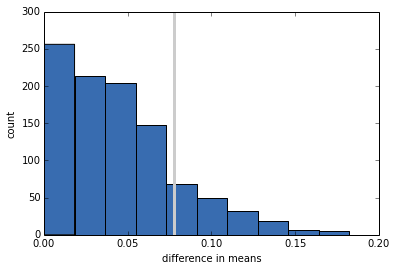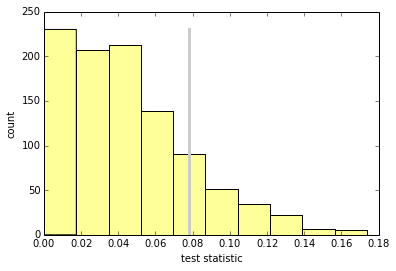Hypothesis Testing¶

Credits: Forked from CompStats by Allen Downey. License: Creative Commons Attribution 4.0 International.

In :
from __future__ import print_function, division

import numpy
import scipy.stats

import matplotlib.pyplot as pyplot

from IPython.html.widgets import interact, fixed
from IPython.html import widgets

import first

# seed the random number generator so we all get the same results
numpy.random.seed(19)

# some nicer colors from http://colorbrewer2.org/
COLOR1 = '#7fc97f'
COLOR2 = '#beaed4'
COLOR3 = '#fdc086'
COLOR4 = '#ffff99'
COLOR5 = '#386cb0'

%matplotlib inline

Part One¶

As an example, let's look at differences between groups. The example I use in Think Stats is first babies compared with others. The first module provides code to read the data into three pandas Dataframes.

In :
live, firsts, others = first.MakeFrames()

The apparent effect we're interested in is the difference in the means. Other examples might include a correlation between variables or a coefficient in a linear regression. The number that quantifies the size of the effect, whatever it is, is the "test statistic".

In :
def TestStatistic(data):
group1, group2 = data
test_stat = abs(group1.mean() - group2.mean())
return test_stat

For the first example, I extract the pregnancy length for first babies and others. The results are pandas Series objects.

In :
group1 = firsts.prglngth
group2 = others.prglngth

The actual difference in the means is 0.078 weeks, which is only 13 hours.

In :
actual = TestStatistic((group1, group2))
actual
Out:
0.078037266777549519

The null hypothesis is that there is no difference between the groups. We can model that by forming a pooled sample that includes first babies and others.

In :
n, m = len(group1), len(group2)
pool = numpy.hstack((group1, group2))

Then we can simulate the null hypothesis by shuffling the pool and dividing it into two groups, using the same sizes as the actual sample.

In :
def RunModel():
numpy.random.shuffle(pool)
data = pool[:n], pool[n:]
return data

The result of running the model is two NumPy arrays with the shuffled pregnancy lengths:

In :
RunModel()
Out:
(array([36, 40, 39, ..., 43, 42, 40]), array([43, 39, 32, ..., 37, 35, 41]))

Then we compute the same test statistic using the simulated data:

In :
TestStatistic(RunModel())
Out:
0.081758440969863955

If we run the model 1000 times and compute the test statistic, we can see how much the test statistic varies under the null hypothesis.

In :
test_stats = numpy.array([TestStatistic(RunModel()) for i in range(1000)])
test_stats.shape
Out:
(1000,)

Here's the sampling distribution of the test statistic under the null hypothesis, with the actual difference in means indicated by a gray line.

In :
def VertLine(x):
"""Draws a vertical line at x."""
pyplot.plot([x, x], [0, 300], linewidth=3, color='0.8')

VertLine(actual)
pyplot.hist(test_stats, color=COLOR5)
pyplot.xlabel('difference in means')
pyplot.ylabel('count')
NoneThe p-value is the probability that the test statistic under the null hypothesis exceeds the actual value.

In :
pvalue = sum(test_stats >= actual) / len(test_stats)
pvalue
Out:
0.14999999999999999

In this case the result is about 15%, which means that even if there is no difference between the groups, it is plausible that we could see a sample difference as big as 0.078 weeks.

We conclude that the apparent effect might be due to chance, so we are not confident that it would appear in the general population, or in another sample from the same population.

Part Two¶

We can take the pieces from the previous section and organize them in a class that represents the structure of a hypothesis test.

In :
class HypothesisTest(object):
"""Represents a hypothesis test."""

def __init__(self, data):
"""Initializes.

data: data in whatever form is relevant
"""
self.data = data
self.MakeModel()
self.actual = self.TestStatistic(data)
self.test_stats = None

def PValue(self, iters=1000):
"""Computes the distribution of the test statistic and p-value.

iters: number of iterations

returns: float p-value
"""
self.test_stats = numpy.array([self.TestStatistic(self.RunModel())
for _ in range(iters)])

count = sum(self.test_stats >= self.actual)
return count / iters

def MaxTestStat(self):
"""Returns the largest test statistic seen during simulations.
"""
return max(self.test_stats)

def PlotHist(self, label=None):
"""Draws a Cdf with vertical lines at the observed test stat.
"""
def VertLine(x):
"""Draws a vertical line at x."""
pyplot.plot([x, x], [0, max(ys)], linewidth=3, color='0.8')

ys, xs, patches = pyplot.hist(ht.test_stats, color=COLOR4)
VertLine(self.actual)
pyplot.xlabel('test statistic')
pyplot.ylabel('count')

def TestStatistic(self, data):
"""Computes the test statistic.

data: data in whatever form is relevant
"""
raise UnimplementedMethodException()

def MakeModel(self):
"""Build a model of the null hypothesis.
"""
pass

def RunModel(self):
"""Run the model of the null hypothesis.

returns: simulated data
"""
raise UnimplementedMethodException()

HypothesisTest is an abstract parent class that encodes the template. Child classes fill in the missing methods. For example, here's the test from the previous section.

In :
class DiffMeansPermute(HypothesisTest):
"""Tests a difference in means by permutation."""

def TestStatistic(self, data):
"""Computes the test statistic.

data: data in whatever form is relevant
"""
group1, group2 = data
test_stat = abs(group1.mean() - group2.mean())
return test_stat

def MakeModel(self):
"""Build a model of the null hypothesis.
"""
group1, group2 = self.data
self.n, self.m = len(group1), len(group2)
self.pool = numpy.hstack((group1, group2))

def RunModel(self):
"""Run the model of the null hypothesis.

returns: simulated data
"""
numpy.random.shuffle(self.pool)
data = self.pool[:self.n], self.pool[self.n:]
return data

Now we can run the test by instantiating a DiffMeansPermute object:

In :
data = (firsts.prglngth, others.prglngth)
ht = DiffMeansPermute(data)
p_value = ht.PValue(iters=1000)
print('\nmeans permute pregnancy length')
print('p-value =', p_value)
print('actual =', ht.actual)
print('ts max =', ht.MaxTestStat())
means permute pregnancy length
p-value = 0.16
actual = 0.0780372667775
ts max = 0.173695697482

And we can plot the sampling distribution of the test statistic under the null hypothesis.

In :
ht.PlotHist()As an exercise, write a class named DiffStdPermute that extends DiffMeansPermute and overrides TestStatistic to compute the difference in standard deviations. Is the difference in standard deviations statistically significant?

In :
class DiffStdPermute(DiffMeansPermute):
"""Tests a difference in means by permutation."""

def TestStatistic(self, data):
"""Computes the test statistic.

data: data in whatever form is relevant
"""
group1, group2 = data
test_stat = abs(group1.std() - group2.std())
return test_stat

data = (firsts.prglngth, others.prglngth)
ht = DiffStdPermute(data)
p_value = ht.PValue(iters=1000)
print('\nstd permute pregnancy length')
print('p-value =', p_value)
print('actual =', ht.actual)
print('ts max =', ht.MaxTestStat())
std permute pregnancy length
p-value = 0.155
actual = 0.176049064229
ts max = 0.44299505029

Now let's run DiffMeansPermute again to see if there is a difference in birth weight between first babies and others.

In :
data = (firsts.totalwgt_lb.dropna(), others.totalwgt_lb.dropna())
ht = DiffMeansPermute(data)
p_value = ht.PValue(iters=1000)
print('\nmeans permute birthweight')
print('p-value =', p_value)
print('actual =', ht.actual)
print('ts max =', ht.MaxTestStat())
means permute birthweight
p-value = 0.0
actual = 0.124761184535
ts max = 0.0917504268392

In this case, after 1000 attempts, we never see a sample difference as big as the observed difference, so we conclude that the apparent effect is unlikely under the null hypothesis. Under normal circumstances, we can also make the inference that the apparent effect is unlikely to be caused by random sampling.

One final note: in this case I would report that the p-value is less than 1/1000 or 0.001. I would not report that p=0, because the apparent effect is not impossible under the null hypothesis; just unlikely.